## Selina Concise Mathematics Class 10 ICSE Solutions Chapter 14 Equation of a Line Ex 14E

These Solutions are part of Selina Concise Mathematics Class 10 ICSE Solutions. Here we have given Selina Concise Mathematics Class 10 ICSE Solutions Chapter 14 Equation of a Line Ex 14E.

Other Exercises

Question 1.
Point P divides the line segment joining the points A (8, 0) and B (16, -8) in the ratio, 3 : 5. Find its co-ordinates of point P. Also, find the equation of the line through P and parallel to 3x + 5y = 7
Solution: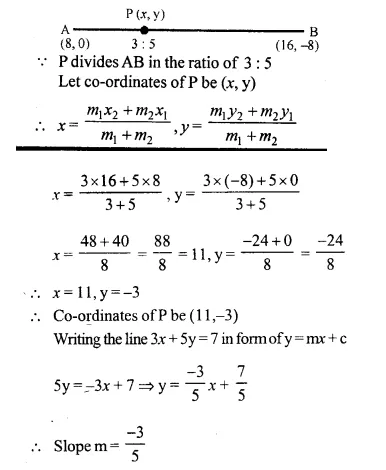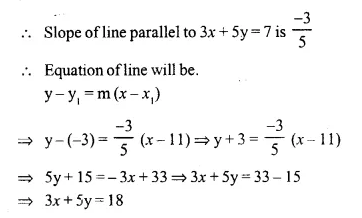Question 2.
The line segment joining the points A (3, -4) and B (-2, 1) is divided in the ratio 1 : 3 at point P in it Find the co-ordinates of P. Also, find the equation of the line through P and perpendicular to the line 5x – 3y = 4.
Solution:
Point P, divides the line segment A (3, -4) and B(-2, 1) in the ratio of 1 : 3
Let co-ordinates of P be (x, y), then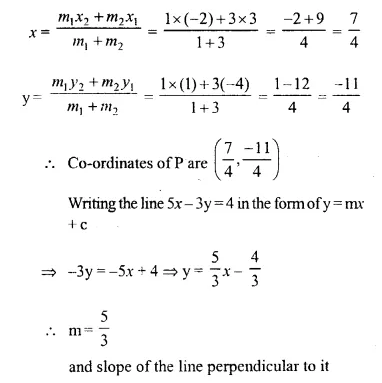Question 3.
A line 5x + 3y + 15 = 0 meets y -axis at point P. Find the co-ordinates of point P. Find the equation of a line through P and perpendicular to x – 3y + 4 = 0.
Solution:
P lies on y-axis and let the co-ordinates of P be (0, y)
P lies also on the line 5x + 3y + 15 = 0 it will satisfy it.
5 x 0 + 3y + 15 = 0
⇒ 3y = -15
⇒ y = -5
Co-ordinates of P are (0, -5)
Now, writing the line x – 3y + 4 = 0 is form of y = mx + c
-3y = -x – 4
⇒ 3y = x + 4
⇒ y = $$\frac { 1 }{ 3 }$$ x + $$\frac { 4 }{ 3 }$$Question 4.
Find the value of k for which the lines kx – 5y + 4 = 0 and 5x – 2y + 5 = 0 are perpendicular to each other. 
Solution:
Writing, the line kx – 5y + 4 = 0 in form of y = mx + c
⇒ -5y = -kx – 4
⇒ 5y = kx + 4Question 5.
A straight line passes through the points P (-1, 4) and Q (5, -2). It intersects the co-ordinate axes at points A and B. M is the mid point of the segment AB. Find:
(i) the equation of the line.
(ii) the co-ordinates of A and B.
(iii) the co-ordinates of M. (2003)Solution: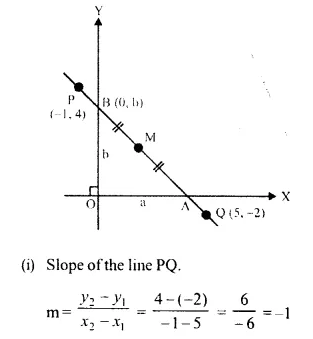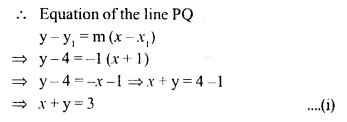(ii) Let. co-ordinates of A be (x, 0) and of B be (0, y) which lie On the line.
Substituting, the co-ordinates in (i)
x + 0 = 3 ⇒x = 3
Co-ordinates of A are (3, 0)
Again 0 + y = 3 ⇒ y = 3
Co-ordinates of B are (0,3)
(iii) M is the mid-point of AB.
Co-ordinates of M wil be ($$\frac { 3 }{ 2 }$$ , $$\frac { 3 }{ 2 }$$)

Question 6.
(1, 5) and (-3, -1) are the co-ordinates of vertices A and C respectively of rhombus ABCD. Find the equations of the diagonals AC and BD.
Solution:
Co-ordinates of A and C of rhombus ABCD are (1, 5) and (-3, -1)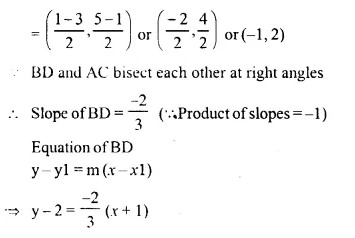⇒ 3y – 6 = -2x – 2
⇒ 2x + 3y = 6 – 2
⇒ 2x + 3y = 4

Question 7.
Show that A (3, 2), B (6, -2) and C (2, -5) can be the vertices of a square.
(i) Find the co-ordinates of its fourth vertex D, if ABCD is a square.
(ii) Without using the co-ordinates of vertex D, find the equation of side AD of the square and also the equation of diagonal BD.
Solution:
Three vertices of a square ABCD are A (3, 2), B (6, -2) and C (2, -5)
Let, co-ordinates of fourth vertex D be (x, y)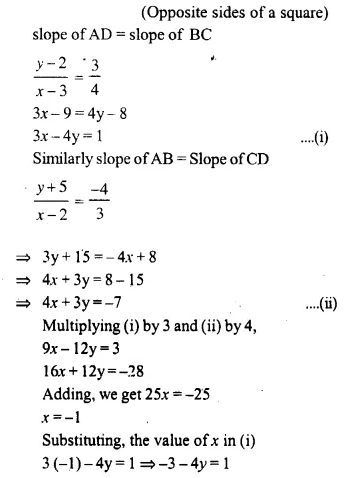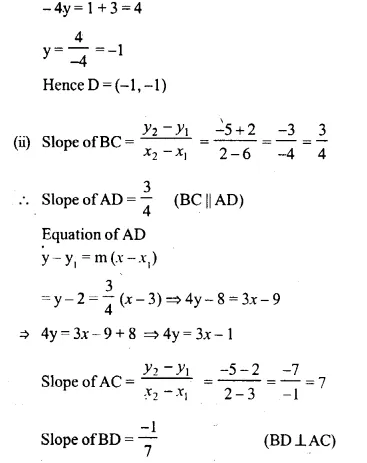Question 8.
A line through origin meets the line x = 3y + 2 at right angles at point X. Find the co-ordinates of X.
Solution:
Slope of line x = 3y + 2 or 3y = x – 2 ….(i)Question 9.
A straight line passes through the point (3, 2) and the portion of this line intercepted between the positive axes, is bisected at this point. Find the equation of the line.
Solution: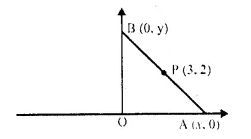Let, the line intersects x-axis at A and y-axis at B.
Let, co-ordinates of A (x, o) and of (o, y)
But (3, 2) is the mid-point of AB.Question 10.
Find the equation of the line passing through the point of intersection of 7x + 6y = 71 and 5x – 8y = -23; and perpendicular to the line 4x -2y = 1.
Solution:
7x + 6y = 71 ….(i)
5x – 8y = -23 ….(ii)
Multiply (i) by 4 and (ii) by 3,
28x + 24y = 284
15x – 24y = -69
On adding (i) and (ii), we get:
43x = 215
x = 5
Substituting, the value of x in (i)
7 x 5 + 6y = 71
35 + 6y = 71
⇒ 6y = 71 – 35 = 36
⇒ y = 6
Point of intersection of these lines is (5, 6)
Now slope of line 4x – 2y = 1
⇒ 4x – 1 = 2y
⇒ y = 2x – $$\frac { 1 }{ 2 }$$ is 2
Slope of line through the point of intersection and perpendicular to 4x – 2y = 1 is $$\frac { -1 }{ 2 }$$
Equation of the line y – y1 = m (x – x1)
⇒ y – 6 = $$\frac { -1 }{ 2 }$$ (x – 5)
⇒ 2y – 12 = -x + 5
⇒ x + 2y = 5 + 12 = 17
⇒ x + 2y = 17

Question 11.
Find the equation of the line which is perpendicular to the line $$\frac { x }{ a }$$ – $$\frac { y }{ b }$$ = 1 at the point where this line meets y-axis.
Solution: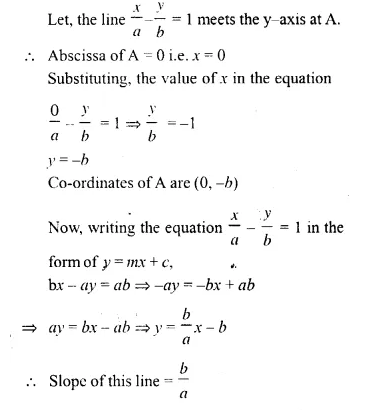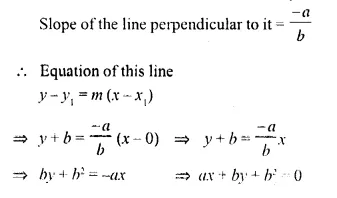Question 12.
O (0, 0), A (3, 5) and B (-5, -3) are the vertices of triangle OAB. Find:
(i) the equation of median of ∆OAB through vertex O.
(ii) the equation of altitude of ∆OAB through vertex B.
Solution:
(i) Let, mid-point of AB be D.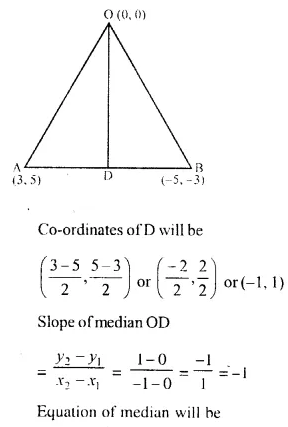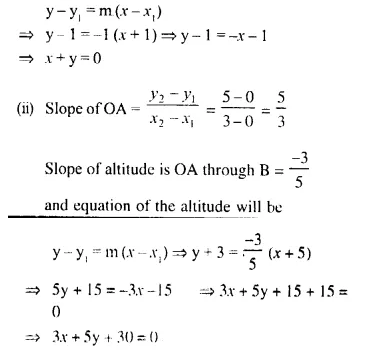Question 13.
Determine whether the line through points (-2, 3) and (4, 1) is perpendicular to the line 3x = y + 1.
Does line 3x = y + 1 bisect the line segment joining the two given points ?
Solution:
Slope of the line joining the points (-2, 3) and (4, 1)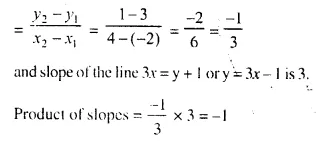Yes, these are perpendicular to each other
Let P be the mid-point of the line joining the points (-2, 3) and (4, 1)
Co-ordinates of P will beThis point (1, 2) satisfies the equaion 3x = y + 1 then, it will bisect the line joining the given point
now, substituting the value of x and y. in 3x = y + 1
⇒ 3 x 1 = 2 + 1
⇒ 3 = 3. which is true.
Yes, the line 3x = y + 1 is the bisector.

Question 14.
Given a straight line x cos 30° + y sin 30° = 2. Determine the equation of the other line which is parallel to it and passes through (4, 3).
Solution:
Equation of the given line is x cos 30° + y sin 30° = 2
y sin 36° = -x cos 30° + 2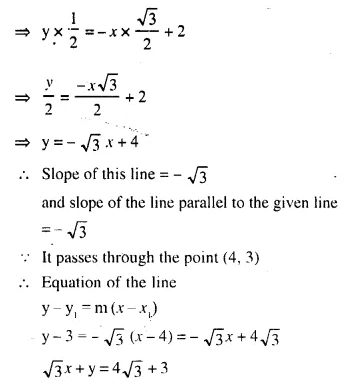Question 15.
Find the value of k such that the line (k – 2) x + (k + 3) y – 5 = 0 is :
(i) perpendicular to the line 2x – y + 7 = 0
(ii) parallel to it.
Solution:
Writing the given equation in the form of y = mx + c
(k – 2) x + (k + 3) y – 5 = 0 ….(i)
⇒ (k + 3) y = – (k – 2) x + 5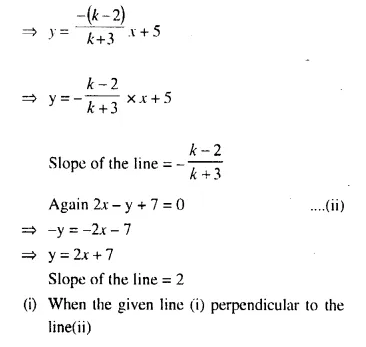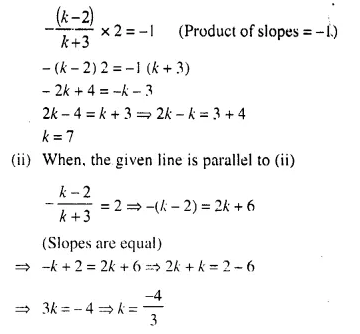Question 16.
The vertices of a triangle ABC are A (0, 5), B (-1, -2) and C (11, 7) write down the equation of BC. Find :
(i) the equation of line through A and perpendicular to BC.
(ii) the co-ordinates of the point P, where the perpendicular through A, as obtained in (i) meets BC.
Solution:
Vertices of ∆ABC are A (0, 5),B (-1, -2) and C (11, 7)(ii) Let, the line through A meets BC in P
P is point of intersection of these two lines.
3x – 4y = 5 ……… (i)
4x + 3y = 15 …….. (ii)
On solving (i), (ii) we get
x = 3, y = 1
Co-ordinates of Pare (3, 1)

Question 17.
From the given figure, find :
(i) the co-ordinates of A, B and C.
(ii) the equation of the line through A and parallel to BC. (2005)Solution:
(i) From the figure, we see that co-ordinates of A are (2, 3), of B are (-1, 2) of C and (3, 0)
(ii) Slope of line BC is (m)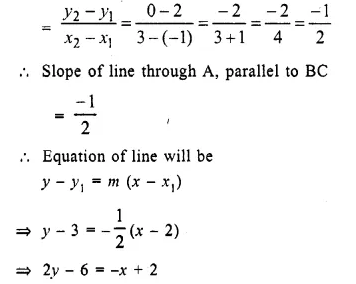⇒ x + 2y – 6 – 2 = 0
⇒ x + 2y – 8 = 0
⇒ x + 2y = 8

Question 18.
P (3, 4), Q (7, -2) and R (-2, -1) are the vertices of triangle PQR. Write down the equation of the median of the triangle through R. (2004)
Solution:
Let (x, y) be the co-ordinates of M, the mid-point of PQ.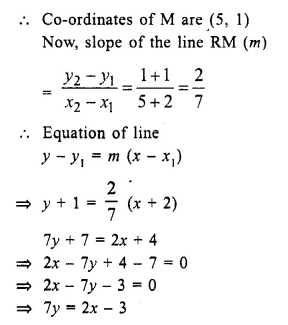⇒ x + 2y – 8 = 0
⇒ x + 2y = 8

Question 19.
A (8, -6), B (-4, 2) and C (0, -10) are the vertjces of a triangle ABC. If P is the mid-point of AR and Q is the mid-point of AC, use co ordinate geometry to show that PQ is parallel to BC. Give a special name to quadrilateral PBCQ.
Solution:
In ∆ABC, co-ordinates of A, B and C are (8, -6), (-4, 2) and C (0, -10) respectively.
P and Q are the mid-points of AB and AC respectively
Co-ordinates of P will be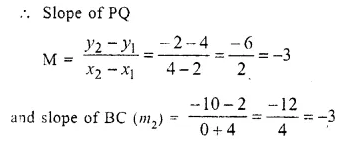Slopes of PQ and BC are same.
These are parallel to each other.

Question 20.
A line AB meets the x-axis at point A and y-axis at point B. The point P(-4, -2) divides the line segment AB internally such that AP : PB = 1 : 2. Find :
(i) the co-ordinates of A and B.
(ii) equation of line through P, and perpendicular to AB.
Solution:
Line AB intersects x-axis at A and y-axis at B.
(i) Let co-ordinates of A be (x, 0) and of B be (0, y)
Point P (-4, -2) intersects AB in the ratio 1 : 2Question 21.
A line intersects x-axis at point (-2, 0) and cuts off an intercept of 3 units from die positive side of y-axis. Find the equation of the line. (1992)
Solution:
Let line intersects x-axis at P (-2, 0) and cuts off an intercept of 3 units at Q.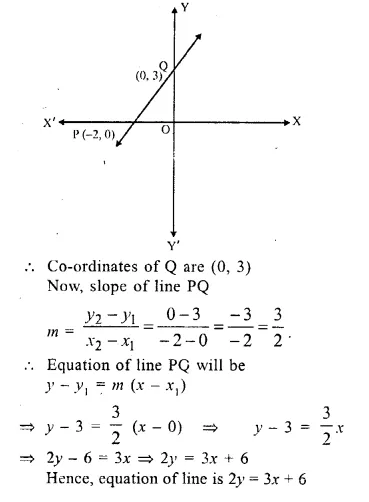Question 22.
Find the equation of a line passing through the point (2, 3) and having the x-intercept of 4 units. (2002)
Solution:
x-intercept = 4
Co-ordinates of that point = (4, 0)
The co-ordinates of the given point (2, 3)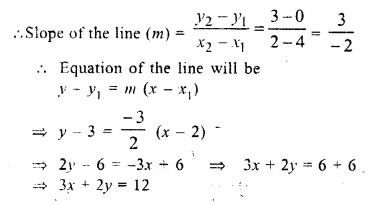Question 23.
The given figure (not drawn to scale) shows two straight lines AB and CD. If’ equation of the line AB is : y = x + 1 and equation of line CD is : y = √3 x – 1. Write down the inclination of lines AB and CD; also, find the angle 6 between AB and CD. (1989)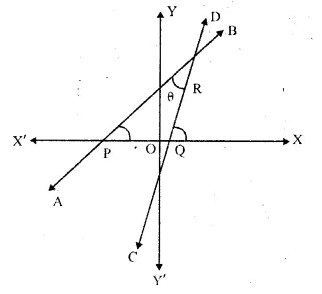Solution:
Equation of line AB is y = x + 1
and equation of line CD is y = √3 x – 1
Slope of AB = 1
tanθ = 1
⇒ θ = 45°
Inclination angles of AB = 45°
Slope of CD = tanθ = √3 = tan 60°
⇒ θ = 60°
Inclination angle of CD = 60°
In ΔPQR,
Ext. ∠RQX = ∠RPQ + ∠PRQ (Exterior angles is equal to sum of its interior opposite angles)
⇒ 60° = 45° + θ
⇒ θ = 60° – 45° = 15°
⇒ θ = 15°

Question 24.
Write down the equation of the line whose gradient is $$\frac { 3 }{ 2 }$$ and which passes through P, where P divides the line segment joining A (-2, 6) and B (3, -4) in the ratio 2 : 3. (1996, 2001)
Solution:
P divides the line segment AB in which A (-2, 6) and B (3, -4) in the ratio 2 : 3
Co-ordinates of P will beQuestion 25.
The ordinate of a point lying on the line joining the points (6, 4) and (7, -5) is -23. Find the co-ordinates of that point.
Solution:
Let points are A (6, 4) and B (7, -5)Question 26.
Points A and B have coordinates (7, -3) and (1, 9) respectively. Find
(i) the slope of AB.
(ii) the equation of the perpendicular bisector of the line segment AB.
(iii) the value of ‘p’ of (-2, p) lies on it.
Solution:
Coordinates of A are (7, -3), of B = ( 1, 9)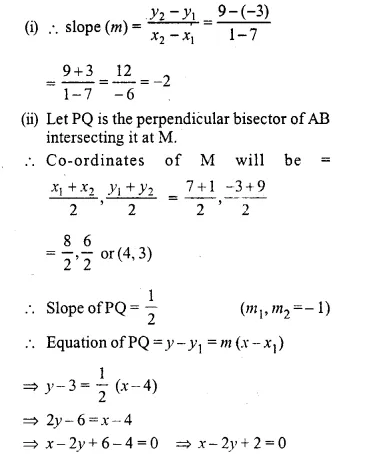Question 27.
A and B are two points on the x-axis and y-axis respectively. P (2, -3) is the mid point of AB. Find the
(i) Coordinates of A and B.
(ii) Slope of line AB.
(iii) equation of line AB.Solution:
As P (2, -3) is mid-point of AB.
Let coordinates of B be (0, y) and coordinates of A be (x, 0)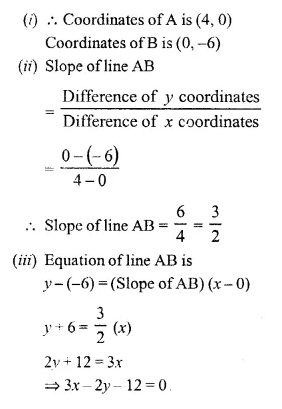Question 28.
The equation of a line is 3x + 4y – 7 = 0. Find:
(i) the slope of the line.
(ii) the equation of a line perpendicular to the given line and passing through the intersection of the lines x – y + 2 = 0 and 3x + y – 10 = 0.
Solution:
Given line 3x + 4y -7 = 0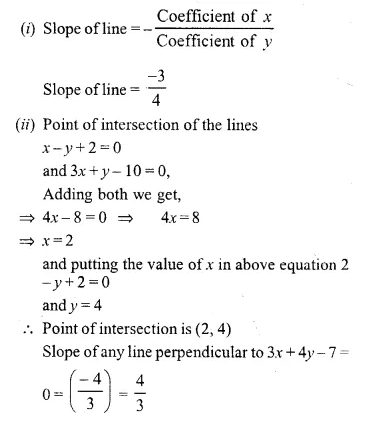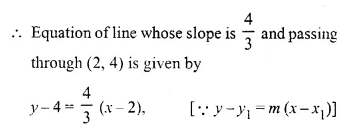⇒ 3 (y – 4) = 4 (x – 2)
⇒ 3y – 12 = 4x – 8
⇒ 4x – 3y – 8 + 12 = 0
⇒ 4x – 3y + 4 = 0

Question 29.
ABCD is a parallelogram where A (x, y), B (5, 8), C (4, 7) and D (2, -4). Find :
(i) co-ordinates of A
(ii) equation of diagonal BD.
Solution:(i) In || gm ABCD, A (x, y), B (5, 8), C (4, 7) and D (2, -4)
The diagonals of ||gm bisect each other
O is said point of AC and BD
Now if O is mid point of BD then its co-ordinates will be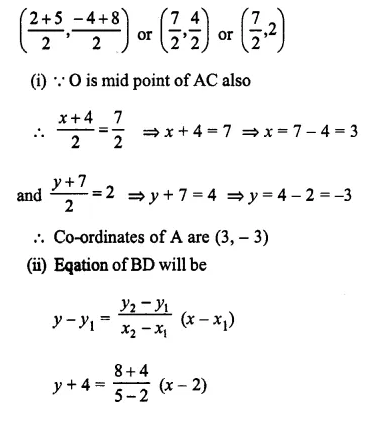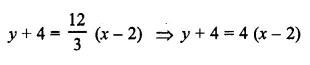⇒ y + 4 = 4x – 8
⇒ 4x – y -8 – 4 = 0
⇒ 4x – y – 12 = 0 or 4x – y = 12

Question 30.
Given equation of line L1 is y = 4.
(i) Write the slope of line L2 if L2 is the bisector of angle O.
(ii) Write the co-ordinates of point P.
(iii) Find the equation of L2.
Solution:
(i) Equation of line L1 is y = 4
L2 is the bisector of ∠O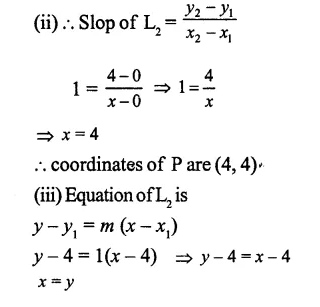Question 31.
Find: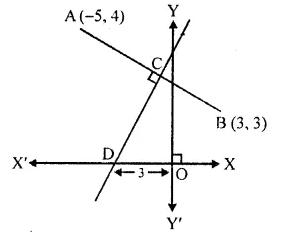(i) equation of AB
(ii) equation of CD
Solution:
Co-ordinates of A and B are (-5, 4) and (3, 3) respectively.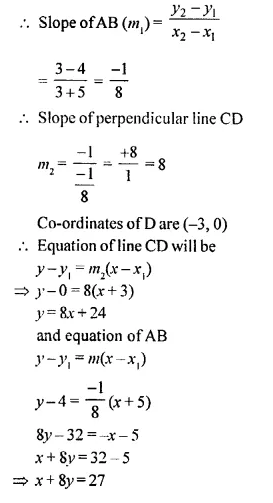Question 32.
Find the equation of the line that has x-intercept = -3 and is perpendicular to 3x + 5y = 1
Solution:
x-intercept of the line = -3
and is perpendicular to the line
3x + 5y = 1
5y = 1 – 3xQuestion 33.
A straight line passes through the points P (-1, 4) and Q (5, -2). It intersects x-axis at point A and y-axis at point B. M is the mid-point of the line segment AB. Find :
(i) the equation of the line.
(ii) the co-ordinates of points A and B.
(iii) the co-ordinates of point M.
Solution:
A line passing through the two points P (-1, 4) and Q (5, -2) intersects x-axis at point A and y- axis at point B.
M is mid-point of AB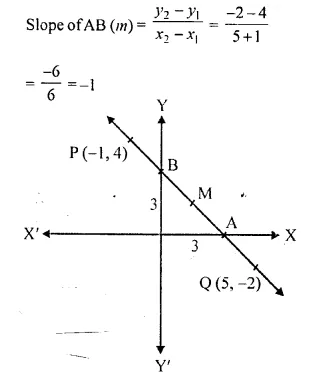(i) Equation of line AB will be
y – y1 = m (x – x1)
⇒ y – 4 = -1 (x + 1)
⇒ y – 4 = – x – 1
⇒ y + x = -1 + 4
⇒ x + y = 3
(ii) The line intersect x-axis at A and OA = 3 units
Co-ordinates of A are (3, 0) and the line intersects y-axis at B and OB = 3 units
Co-ordinates of B are (0, 3)
(iii) M is mid-point of AB
Co-ordinates of M are ($$\frac { 3 }{ 2 }$$ , $$\frac { 3 }{ 2 }$$)

Question 34.
In the given figure, line AB meets y-axis at point A. Line through C (2, 10) and D intersects line AB at right angle at point P. Find: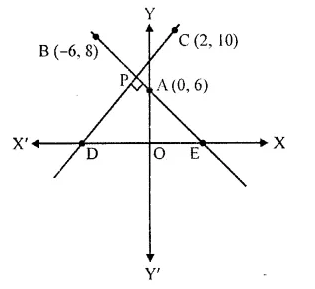(i) equation of line AB.
(ii) equation of line CD.
(iii) co-ordinates of points E and D.
Solution:
In the given figure, AB meets y-axis at point A.
Line through C (2, 10) and D intersects line AB at P at right angle.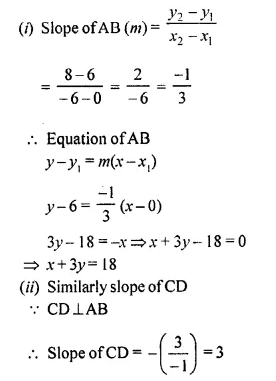Equation of CD
y – 10 = 3 (x – 2)
⇒ y – 10 = 3x – 6
⇒ 3x – y + 10 – 6 = 0
⇒ 3x – y + 4 = 0
(iii) Co-ordinates of D which is on x-axis
3x – y + 4 = 0
3x – 0 + 4 = 0
⇒ 3x + 4 = 0
⇒ 3x = -4
⇒ x = $$\frac { -4 }{ 3 }$$
Co-ordinates of D are ($$\frac { -4 }{ 3 }$$ , 0)
E is also on x-axis
x + 3y = 18
Substituting, y = 0, then
x + 0= 18
⇒ x = 18
Co-ordinates of E are (18, 6)

Question 35.
A line through point P (4, 3) meets x-axis at point A and the y-axis at point B. If BP is double of PA, find the equation of AB.
Solution:
A line through P (4, 3) meets x-axis at A and the y-axis at B. If BP is double of PA.
Draw BC || x-axis
and PC || y-axis
∠P = ∠P (common)
∠D = ∠C (each 90°)
PB = 2PA ⇒ PA = $$\frac { 1 }{ 2 }$$ PB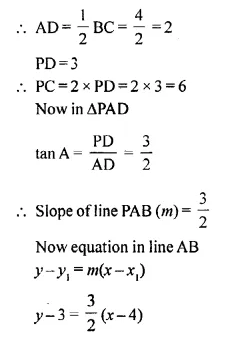2y – 6 = 3x – 12
⇒ 3x – 2y – 12 + 6 = 0
⇒ 3x – 2y – 6 = 0
⇒ 3x – 2y = 6

Question 36.
Find the equation of line through the intersection of lines 2x – y = 1 and 3x + 2y = -9 and making an angle of 30° with positive direction of x-axis.
Solution:
Equation of given two intersecting lines are 2x – y = 1 and 3x + 2y = -9 Which make an angle of 30°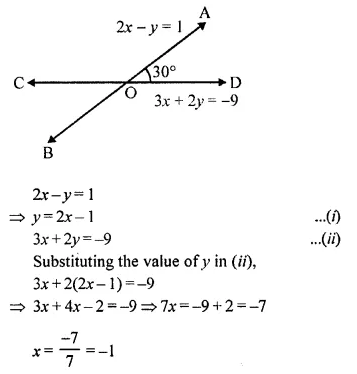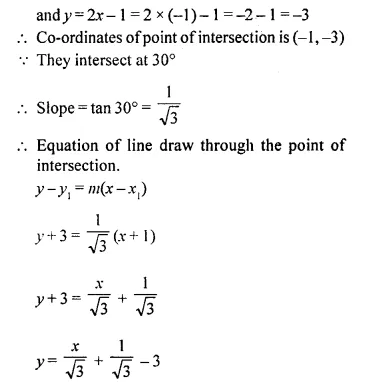Question 37.
Find the equation of the line through the points A (-1, 3) and B (0, 2). Hence, show that the points A, B and C (1, 1) are collinear.
Solution:
The given points are A (-1, 3) and B (0, 2) and co-ordinates of a point C are (1, 1)
Now slope of the line joining A and BEquation of the line y – y1 = m(x – x1)
⇒ y – 2 = -1 (x – 0)
⇒ y – 2 = -x
⇒ x + y – 2 = 0
Point C (1, 1) will be on AB if it satisfy
1 + 1 – 2 = 0
⇒ 0 = 0
Point C lies on AB
Hence points A, C and B are collinear.

Question 38.
Three vertices of a parallelogram ABCD taken in order are A (3, 6), B (5, 10) and C (3, 2), find:
(i) the co-ordinates of the fourth vertex D.
(ii) length of diagonal BD.
(iii) equation of side AB of the parallelogram ABCD. (2015)
Solution:
Three vertices of a ||gm ABCD taken an order are A (3, 6), B (5, 10) and C (3, 2)
Join diagonals AC and BD which bisect each other at O.
O is mid-point of AC as well as of BD
Now co-ordinates of O will be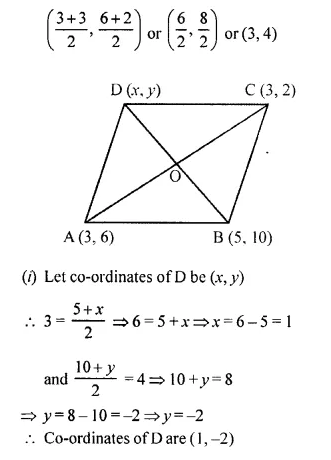Question 39.
In the figure, given, ABC is a triangle and BC is parallel to the y-axis. AB and AC intersect the y-axis at P and Q respectively.(i) Write the co-ordinates of A.
(ii) Find the length of AB and AC.
(iii) Find the ratio in which Q divides AC.
(iv) Find the equation of the line AC. (2015)
Solution:
In the given figure,
ABC is a triangle and BC || y-axis
AB and AC intersect the y-axis at P and Q respectively.
(i) Co-ordinates of A are (4,0).
(ii) Length of AB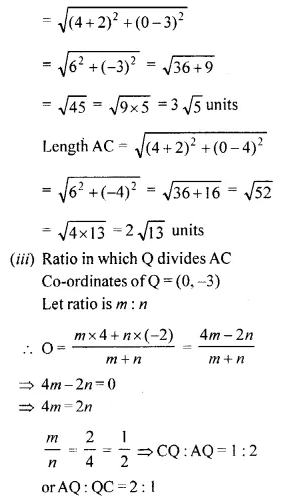Question 40.
The slope of a line joining P (6, k) and Q (1 – 3k, 3) is $$\frac { 1 }{ 2 }$$. Find :
(i) k
(ii) mid-point of PQ, using the value of ‘A’ found in (i). (2016)
Solution:
(i) Slope of the line joining P(6, k) and Q (1 –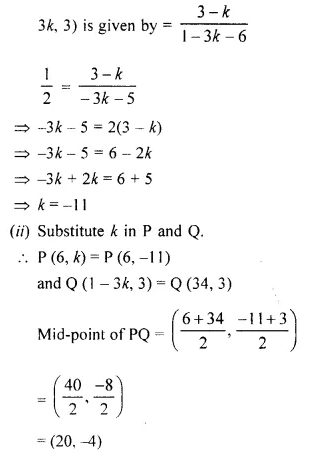Question 41.
A line AB meets X-axis at A and Y-axis at B. P (4, -1) divides AB in the ratio 1 : 2.
(i) Find the coordinates of A and B.
(ii) Find the equation of the line through P and perpendicular to AB.Solution:
(i) Since, A lies on the x-axis,
let the coordinates of A be (x, 0).
Since B lies on the y-axis,
let the coordinates of B be (0, y).
Let m = 1 and n = 2.
Using section formula,Hope given Selina Concise Mathematics Class 10 ICSE Solutions Chapter 14 Equation of a Line Ex 14E are helpful to complete your math homework.

If you have any doubts, please comment below. Learn Insta try to provide online math tutoring for you.